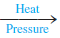# Problem: Urea (H2NCONH2) is used extensively as a nitrogen source in fertilizers. It is produced commercially from the reaction of ammonia and carbon dioxide:2NH3(g) + CO2(g)  H2NCONH2(s) + H2O(g)Ammonia gas at 223°C and 90. atm flows into a reactor at a rate of 500. L/min. Carbon dioxide at 223°C and 45 atm flows into the reactor at a rate of 600. L/min. What mass of urea is produced per minute by this reaction assuming 100% yield?

###### FREE Expert Solution

We are asked to calculate the mass of urea is produced per minute by this reaction assuming 100% yield

2NH3(g) + CO2(g) →  H2NCONH2(s) + H2O(g)

Step 1. Calculate moles per minute of each

NH3

n = 1105.27 mol/min NH3

CO2

n = 663.16 mol/min CO2

Step 2. Simultaneously calculate the theoretical yield and identify the limiting reactant.

Use both to calculate for H2NCONH2

Molar mass H2NCONH= 60.07 g/mol

88% (258 ratings)###### Problem Details

Urea (H2NCONH2) is used extensively as a nitrogen source in fertilizers. It is produced commercially from the reaction of ammonia and carbon dioxide:

2NH3(g) + CO2(gH2NCONH2(s) + H2O(g)

Ammonia gas at 223°C and 90. atm flows into a reactor at a rate of 500. L/min. Carbon dioxide at 223°C and 45 atm flows into the reactor at a rate of 600. L/min. What mass of urea is produced per minute by this reaction assuming 100% yield?

Frequently Asked Questions

What scientific concept do you need to know in order to solve this problem?

Our tutors have indicated that to solve this problem you will need to apply the Gas Stoichiometry concept. You can view video lessons to learn Gas Stoichiometry. Or if you need more Gas Stoichiometry practice, you can also practice Gas Stoichiometry practice problems.

What professor is this problem relevant for?

Based on our data, we think this problem is relevant for Professor Velazquez Mojica's class at UCD.

What textbook is this problem found in?

Our data indicates that this problem or a close variation was asked in Chemistry: An Atoms First Approach - Zumdahl Atoms 1st 2nd Edition. You can also practice Chemistry: An Atoms First Approach - Zumdahl Atoms 1st 2nd Edition practice problems.